# A Simple Intuition Behind The Normal Distribution Equation

Ever since I learned about the Normal Distribution Equation in school it always looked menacing to me. I could not memorize it and I could not make any sense of it. I can kind of understand why the equation includes the sigma, mean, and the x’s. But where did e come from? Pi? And these strange fractions everywhere.

In this article we are going to derive the equation for the normal distribution (pdf) from scratch, step-by-step, using simple examples. I guarantee you by the end of this article you will be able to do it on your own with minimal algebra and probability knowledge required.

The full code is available on my GitHub. The resources that were used in preparation for this article are: , , , .

# 1. From Darts to Exponents

Let’s imagine we are throwing darts at a dartboard. As we do this, we record each dart’s position on the board. Below is a scatter plot of the final result, where the bullseye is centered on the origin (0, 0).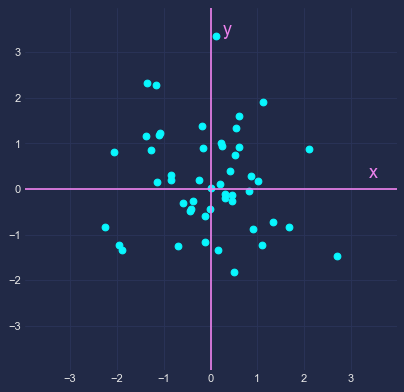The positions of darts after 50 throws

As we can expect, most of the darts on the board cluster in the close proximity to the desired target. As we move further away from the bullseye, fewer and fewer darts fall there. How can we come up with a function to calculate a probability of a dart in a particular location on the board? In other words, how can we define a pdf that would take a location on the board and produce a probability of a dart being there?

Let’s define a pdf called phi that would take a point on the dartboard and produce a probability of finding a dart near that point. We are going to define a small box dA to describe how near to that point we want to find the darts.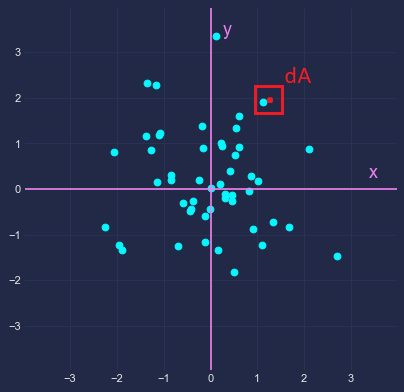The point is the red dot, with the square dA surrounding it

Then, the probability of finding a dart inside that box can be described by the following formula: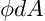The coordinates of the red dot plugged into phi multiplied by the area dA

Please note that boxes that lie closer to the origin will produce higher probability, as it naturally follows from our assumptions that there are more darts closer to the bullseye. Also, if two boxes are the same distance away from the origin, then the larger box will have a higher probability, since it has a larger area.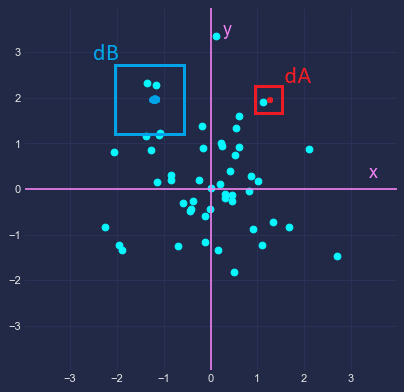dB and dA are equally distant from the origin, but dB is larger

You might have noticed that I use the word distance as opposed to specific coordinates of x and y. This is because y and x are independent of each other: knowing one does not tell you anything about the other. If you aim your dart too high, say at y=2.5, then it does not tell you anything about which side of the board (i.e., x) you are going to hit. It follows that if you rotate a box dA preserving it’s distance r and its area dA, you will get the same value from phi.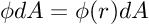phi is rotationally symmetric, with distance r

Let’s define a new pdf f(x) that would take a coordinate x and produce the probability of a dart at that coordinate. Rewriting the previous formula, we get

where f can be thought of some pdf independently operating on the x and y coordinates. Since x and y are independent, we can safely multiply them like this, following the probability independence rule. By cancelling out dA’s we get the following:

Recall, that by the Pythagorean theorem, in the right triangle, the hypothenuse equals the squared root of the squared sum of two legs. Graphically, this can be depicted like so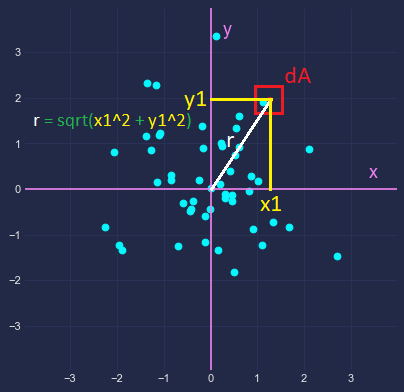r is the hypothenuse, and (0,x1), (0,y1) are two legs of a right triangle

Using this information, we can rewrite the previous equation like this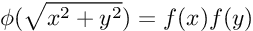r is replaced by the Pythagorean theorem

Let’s set y=0 and plug it into our equation

Since f(0) is some unknown constant, let’s name it lambda

The resultant equation tells us that the function phi operating on some input x is a scalar multiple of f operating on the same input. Using this property, let’s rewrite the previous equation like so

In other words,

Let’s divide both sides by lambda squared and simplify a bit

Substitute

Our goal now is to find a certain function g that would satisfy the property above. That is, if we take a function g and apply it on the x input times the y input we should get a squared root of the sum of squares of those x and y inputs. To find such a function, let’s consider a simpler case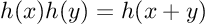Notice the lack of the square root and the squared x and y

What kind of functions have this property? Recalling High School algebra, we can notice that exponentiation functions satisfy the property above. For example,

Although the function h looks similar to the function g, they are not exactly the same. The function g has a squared root in the right hand side of the equation. Therefore, to get rid of it, we can square our inputs of the function g. Squaring x gives us , squaring y gives us , and squaring sqrt(x²+y²) gives us x²+y². We are ready to substitute these values back

You might notice that we chose the base 5 for our formula. In fact, we can choose any base, like 1, 2 or e. To generalize over all the bases, let’s pick the base of e^A, where A is some unknown constant.

Substituting back into g we finally get the following

# 2. A “Normal Distribution” with Two Parameters

Let’s plot the resultant distribution using various values for the lambda and A

Oh no, our distribution is upside down. So let’s fix that by substituting A for the following: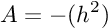Notice how we square first and then apply the negative sign

We get the following equation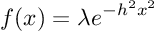The negative term h² mirrors the distribution

Now, by plotting our distributions with various parameters of lambda and h we can observe how to shape of the distribution changes depending on the values of lambda and h.

# 3. From Two to One

So far so good, the curve looks like a normal distribution. However, one problem still persists: for this curve to be considered a true pdf, the area under our curve should be equal to 1. Mathematically,

Let’s solve this integral. Substituting our equation we get

The resultant integral is called Gaussian integral and is equal to the square root of pi. I don’t know why that is, but the complete derivation can be looked up on the Wikipedia. So we get

Let’s substitute in our original equation we derived earlier. We finally get

There are 2 important things we did. First, we got rid of the unknown h, leaving only a single unknown — lambda. Second, since we computed the integral equals 1, we are now guaranteed to have the area under the curve equal to 1. By constraining ourselves with a single parameter lambda, we can see how, unlike our previous examples, the width of the distribution now depends on the value of lambda.

# 4. From One to Spread

What lambda essentially controls is the spread of the values (variance- sigma²). The higher the value — the lower the spread — the narrower the distribution is. Let’s define that relationship between lambda and sigma mathematically.

We know that the variance of a pdf is calculated by the following formula:

Substituting for the f(x) gives us

Now we can clearly see that lambda and sigma are inversely proportional: the higher the lambda — the lower the sigma, and vice versa.

Performing the final substitution for lambda we get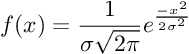Notice how it looks almost like the actual equation already

# 5. The Final Form: Spread and Mean

The formula above describes a normal distribution that is centered on 0. That is, the mean is 0. Suppose we want to be able to center it on different values. From the perspective of the distribution, it means shifting it along the x-axis to the right (positive mean) and to the left (negative mean).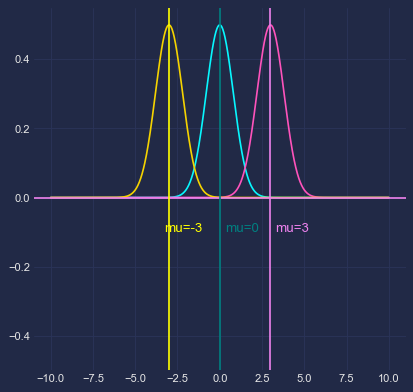3 normal distributions with different means and the same variance

In other words, we need to add (substract) a constant value mu to (from) each of the x’s. As the previous equation shows, there is only one x in that equation, so we simply modify it like soNotice the top right part where mu is applied to x

Recall that to move a curve on the x-axis to the right we need to subtract the constant, and to move it to the left, we need to add it. Since we want positive means to be on the right, we substract it from the x’s.

And this is it, we have finally arrived at the final equation for the normal distribution. I hope this article helped you to gain a bit of intuition behind the normal distribution formula!

--

--